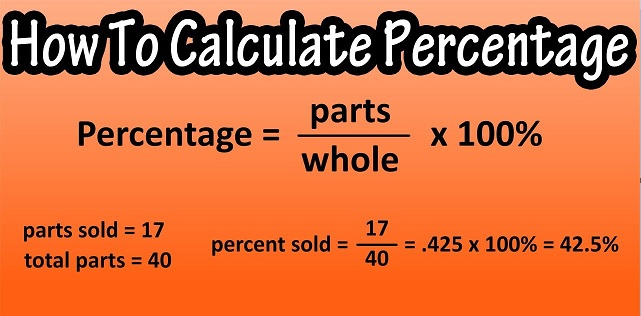# How To Find Percentage (Calculate Formula and Tricks)

Percentage is a complex mathematical word that can easily be misunderstood. In this blog post, we will provide you with a step-by-step guide on how to find percentage without a calculator. This guide will help you understand percentage concepts and apply them to your own life.

Main Points

### How To Find Percentage

Percentage is a calculation that tells how much of a number is represented by a certain percentage. Percentage can be found using different methods, but the easiest way is to use a calculator. To find the percentage, first divide the number by 100. Next, multiply the result by 100 to get the percentage.

### What is Percentage?

Percentage is a number that represents the amount of something out of 100. Percentage can be used in calculations to find proportions, sizes, and other information.

## How To Find Percentage (Calculate Formula and Tricks)### How to Calculate Percentage

Percentage can be calculated by dividing a number by 100. To find the percentage, you first need to divide the number by the total.
For example, if you have a number that is 100% and you want to find its percentage, divide it by the total.

### Percentage Formula

To determine the percentage, we have to divide the value by the total value and then multiply the resultant by 100.

Percentage formula = (Value/Total value) × 100

Example: 2/5 × 100 = 0.4 × 100 = 40 per cent

## How to calculate the percentage of a number?

To calculate the percentage of a number, we need to use a different formula such as:

P% of Number = X

where X is the required percentage.

If we remove the % sign, then we need to express the above formulas as;

P/100 * Number = X

Example: Calculate 10% of 80.

Let 10% of 80 = X

10/100 * 80 = X

X = 8

Conclusion

In this article, we will show you how to find the percentage of a number. Percentage is a very important concept that is used in many different fields, such as accounting and mathematics. To calculate the percentage of a number, you first need to know its base value. Then, you need to divide the base value by the total number. Lastly, you need to multiply the result by 100%.

error: Content is protected !!